# Environmental Health - Calculating Paint Needed

## Problem:

A rectangular swimming pool, with a width of 6.1 m and the cross section as shown in the diagram below, is to be lined with epoxy paint. If a minimum dry coat thickness of 3.0 mm is required and the shrinkage upon drying is 25%, how many litres of paint are needed?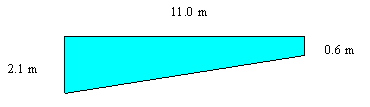## Solution:

The pool has two trapezoidal sides as shown above. (Called Area 1).

The pool water on the top is flat, so each end wall will be rectangular.

Area 2 = "deep end" end wall which will be 2.1 x 6.1 m

Area 3 = "shallow end" which will be 0.6 x 6.1 m

Area 4 = the bottom of the pool. If you look down at it from above the pool you will see it is a rectangle which is 6.1 m wide. We will get its length by creating a right angle triangle in the diagram above. The left side of the triangle will have length 2.1-0.6 = 1.5 m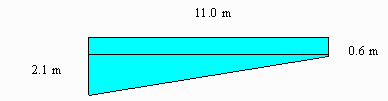The Interior Surface Area will consist of:

(Area 1 x 2) + (Area 2) + (Area 3) + (Area 4)

Area 1 = trapezoid: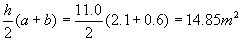Area 2 = Rectangle: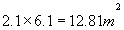Area 3 = Rectangle: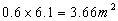Find the length of the angled bottom wall of the pool by using the Pythagorean Theorem.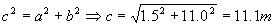Area 4 = Rectangle: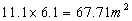Total Area: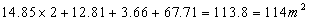Imagine laying all the pieces out flat. You want these covered uniformly with a very thin layer of epoxy paint. In this case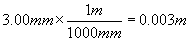Thus the volume of paint required before shrinkage allotment.

Volume=Area x thickness: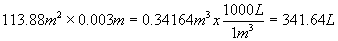Since the paint shrinks by 25% of what you start with, then 75% of the total required = 341.64L

Total Paint Required: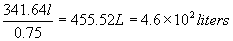Written by Louise Routledge, June 26, 1998